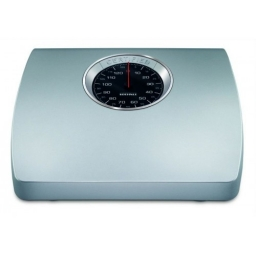# Even-handed 21293

In the eighth grade, the children gathered up 850 kg of paper. Class 8A collected 50 kilograms more than 8B. Class 8 C is even-handed in 200% of what class 8A is. How much paper did each class collect?

a =  225
b =  175
c =  450

### Step-by-step explanation:

a+b+c=850
a = 50+b
c = 2a

a+b+c=850
a = 50+b
c = 2·a

a+b+c = 850
a-b = 50
2a-c = 0

Pivot: Row 1 ↔ Row 3
2a-c = 0
a-b = 50
a+b+c = 850

Row 2 - 1/2 · Row 1 → Row 2
2a-c = 0
-b+0.5c = 50
a+b+c = 850

Row 3 - 1/2 · Row 1 → Row 3
2a-c = 0
-b+0.5c = 50
b+1.5c = 850

Row 3 + Row 2 → Row 3
2a-c = 0
-b+0.5c = 50
2c = 900

c = 900/2 = 450
b = 50-0.5c/-1 = 50-0.5 · 450/-1 = 175
a = 0+c/2 = 0+450/2 = 225

a = 225
b = 175
c = 450

Our linear equations calculator calculates it.Did you find an error or inaccuracy? Feel free to write us. Thank you!

Tips for related online calculators